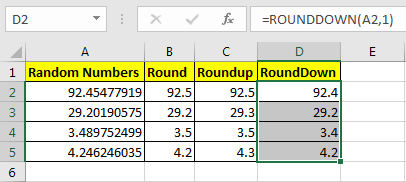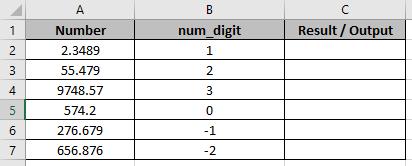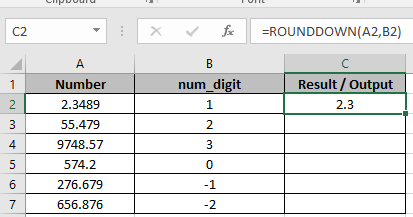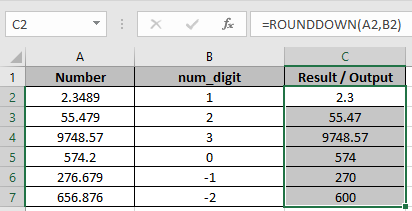# How to use the ROUNDDOWN function in ExcelIn this article, we will learn How to use the ROUNDDOWN function in Excel.

What is the ROUND function used for ?

While playing with big numbers, all digits of a number doesn’t matter that much and we can ignore them to make it more readable and relatable. For example, the 1225411 number is more readable when we write it as 1225000. This is called rounding. There are three popular functions used for rounding up or down functions.

ROUND function rounds down the number depending upon the rounding digit. Less than 4 or equals to 4 will be rounded down and greater than 4 are rounded up. For example 54 will be rounded to 50 whereas 55 will be rounded to 60

ROUNDUP function rounds up the number and doesn't care about the rounding digit. For example 53, 56, 59 will be rounded up to 60.

ROUNDDOWN function rounds down the number and doesn't care about the rounding digit. For example 53, 56, 59 will be rounded down to 50.

ROUNDDOWN function in Excel

ROUNDDOWN function rounds DOWN the numbers upto given rounding digit position. Unlike ROUNDUP function, which rounds up the number.

ROUNDDOWN function Syntax:

 =ROUNDDOWN( number, num_digit )

number : The Number to round off

num_digit : Up to digit, we need to round off.

Example :

All of these might be confusing to understand. Let's understand how to use the function using an example.

The ROUNDDOWN function just shows the last decimal digit as it is and makes all following decimal digits 0. In short, it prevents the rounding up of a number.

In Cell D2 I have written this formula:

 =ROUNDOWN(A2,1)

You can see that 92.454779 is now 92.4. Similarly, all numbers in column D are shown as they depend on defined decimal digits.Pro Note: This does not decrease the last digit.

Now you know how to harness control of these decimal digits to your desired number digits. I tried to explain

Alternate method

ROUNDDOWN function with variable num_digit

In one Column there are Numbers and in other, there are num_digits up to which the number to be rounded down.In one Column there are Numbers and in other, there are num_digits up to which the number to be rounded down.

Use the formula in C2 cell.

 =ROUNDDOWN(A2,B2)

Explanation:

Here in C2 cell, the number will be round off to 1 decimal place.As you can see 2.4 is rounded DOWN to 1 decimal place.

Copy the formula using Ctrl + D taking the first cell till the last cell needed formula.As you can see

1.  First 3 cells are rounded down upto 1, 2 and 3 decimal places respectively.
2. In C5, The number is rounded down to the nearest whole number.
3. The last 2 cells are rounded down to the nearest 10 and 100 respectively.
 To get the number rounded down upto the nearest whole number, 0 is used default as num_digit

Here are all the observational notes using the ROUNDDOWN function in Excel
Notes :

1. The formula only works with numbers.
2. The formula returns the nearest last multiple given input any decimal number.
3. Negative significance is only allowed when number itself is negative else the formula returns #NUM! error.
4. Blank cell or 0 as significance value returns #DIV/0! error.
5. The function returns #VALUE! Error if the date or time value is invalid.
6. Excel stores time value as numbers. So check the format of cell while operating this formula.

Hope this article about How to use the ROUNDDOWN function in Excel is explanatory. Find more articles on calculating values and related Excel formulas here. If you liked our blogs, share it with your friends on Facebook. And also you can follow us on Twitter and Facebook. We would love to hear from you, do let us know how we can improve, complement or innovate our work and make it better for you. Write to us at info@exceltip.com.

Related Articles :

How to use the FLOOR function in Excel | rounds down the number to the nearest specified multiple using the FLOOR function in Excel

How to use the ROUND function in Excel | Rounds off the given number to the nearest num_digit decimal using the ROUND function in Excel

How to use the ROUNDUP function in Excel | Rounds up the given number to the nearest num_digit decimal using the ROUNDUP function in Excel

How to use the ROUNDDOWN function in Excel | Rounds down the given number to the nearest num_digit decimal using the ROUNDDOWN function in Excel

How to use the MROUND function in Excel | rounds off the number to the nearest specified multiple using the MROUND function in Excel.

Popular Articles :

How to use the IF Function in Excel : The IF statement in Excel checks the condition and returns a specific value if the condition is TRUE or returns another specific value if FALSE.

How to use the VLOOKUP Function in Excel : This is one of the most used and popular functions of excel that is used to lookup value from different ranges and sheets.

How to use the SUMIF Function in Excel : This is another dashboard essential function. This helps you sum up values on specific conditions.

How to use the COUNTIF Function in Excel : Count values with conditions using this amazing function. You don't need to filter your data to count specific values. Countif function is essential to prepare your dashboard.

Terms and Conditions of use

The applications/code on this site are distributed as is and without warranties or liability. In no event shall the owner of the copyrights, or the authors of the applications/code be liable for any loss of profit, any problems or any damage resulting from the use or evaluation of the applications/code.